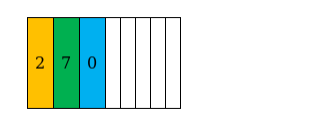# How many 8-digit telephone numbers can be constructed using the digits 0

Question:

How many 8-digit telephone numbers can be constructed using the digits 0 to 9 if each number starts with 270 and n digit appears more than once?

Solution:

Given:8 digit telephone number starts with 270 .

To find: How many 8-digit telephone numbers can be constructed?There are 10 digits between 0 to 9,and three of them are utilized in filling up the first three digits i.e.270 of the 8 digit phone number, so remaining number of digits=10-

$3=7$, and this need to be used in filling up the remaining $8-3=5$ places of the telephone number.

i.e. the remaining 5 places need to filled up with any one of:1,3,4,5,6,8,9

So, number of ways $=7 \times 6 \times 5 \times 4 \times 3=2520$.